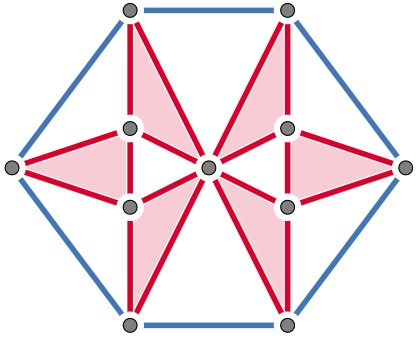# LSE Winter Workshop in Combinatorics## General information

This is a small problem-solving workshop that runs Mon 12 Decemper - Fri 16 December 2022. The focus is on Extremal and Probabilistic Combinatorics. Participation is by invitation only.

We thank the Mathematics Department of the LSE for their financial support.

Organisers

Location

The workshop takes place on the second floor of Columbia House at LSE, which is on the Aldwych.

The talks and coffe breaks will be in COL 2.01 (besides the Thursday public talk, which is in PAR.2.03).

## Timetable

Monday

• 09:30 Coffee, Tea & Fruit
• 10:15 Talk: Nora Frankl
• 11:00 Problem Session
• 12:30 Lunch
• 13:30 Coffee & Tea
• 14:00 Group Work
• 17:00 Wine & Cheese

Tuesday

• 09:30 Coffee, Tea & Fruit
• 11:00 Talk: Luke Collins
• 12:00 Lunch
• 13:00 Coffee & Tea
• 13:30 Group Work
• 15:30 Cake
• 16:00 Group Work (till 17:00)

Wednesday

• 09:30 Coffee, Tea & Fruit
• 10:00 Talk: Matías Pavez-Signé
• 11:00 Group Work
• 12:00 Lunch
• 13:00 Coffee & Tea
• 13:30 Group Work
• 16:00 Cake
• 16:30 Progress Meeting
• 18:00 Workshop Dinner

Thursday

Friday

• 09:30 Coffee, Tea & Fruit
• 10:00 Talk: Domenico Mergoni
• 11:00 Group Work
• 13:00 Pizza & Wine
• 14:00 Progress Meeting

# Talks

Elad Aigner-Horev: Complete minors in randomly perturbed graphs

The theory of Random Graphs asserts that Hadwiger’s conjecture holds true for most graphs and in a rather strong sense at that; the Hadwiger number (size of the largest complete minor) of a random graph typically dominates its chromatic number. The following is then a natural question: What is the least $$p:=p(n)$$ such that given any (sufficiently large) n-vertex graph $$G$$ if one “sprinkles” $$pn^2$$ independent random edges over $$G$$, then the resulting graph a.a.s. satisfies Hadwiger’s conjecture? Hadwiger’s conjecture stipulates that $$p = 0$$ is sufficient and is currently an outstanding conjecture in Combinatorics. The above question proposes a certain mitigation over this conjecture and prompts interest in studying the Hadwiger number of randomly perturbed graphs. In this talk, we present our results pertaining to the emergence of large complete minors and large complete topological minors in randomly perturbed graphs. If time permits, then we shall also mention some of the results we have concerning the diameter and vertex-connectivity of randomly perturbed graphs. Joint work with Dan Hefetz and Michael Krivelevich.

Luke Collins: The Canonical Double Cover of a Graph

The CDC of a graph $$G$$ is its direct product with $$K_2$$. Given two graphs $$G$$ and $$H$$, what can we say if we know that CDC$$(G)\simeq$$ CDC$$(H)$$? In this talk, we give an equivalent characterisation of this property, and observe some of the structural invariants it forces on the base graphs, including their degree sequence, number of walks of any length, and their walk colouring.

Nora Frankl: Ramsey distances on a circle

We call a $$k$$-tuple of distances $$(d_1,...,d_k)$$ Ramsey, if $$d_1+...+d_k=1$$ and any red-blue colouring of a circle with unit perimeter contains a monochromatic k-tuple $$(p_1,...,p_k)$$ such that $$\{|p_i-p_{i+1}|: 1<=i<=k\}=\{d_1,...,d_k\}$$. We characterise Ramse tuples for $$k<8$$, and show that for any k there is at most one Ramsey tuple up to permutation. We also connect this problem to a number theory conjecture, and consider a robust version. Joint work with Gábor Damásdi, János Pach and Dömötör Pálvölgyi.

Domenico Mergoni: Graphs with large minimum degree and no small odd cycles are three colourable

We prove that for $$k$$ large any $$n$$-vertex graph $$G$$ with minimum degree at least $$\frac{1}{2k-1}n$$ without odd cycles of length less than $$2k+1$$ is $$3$$-colourable.

Matías Pavez-Signé: Minimum codegree conditions forcing spanning hypergraphs

Since the classical result of Dirac from 1952 about Hamilton cycles, there have been enormous efforts to find sufficient degree conditions forcing the containment of other spanning structures, such as powers of Hamilton cycles, trees of bounded degrees, graphs with sublinear bandwidth, etc. In this talk, we will study extensions of such results to k-uniform hypergraphs, in particular, hypergraph trees and powers of tight cycles.

Katherine Staden: Counting subgraphs (public talk in PAR.2.03)

Given a small graph $$F$$, how many copies of $$F$$ can a large host graph $$G$$ contain? What is the extremal behaviour – the maximum and minimum number of copies – among all host graphs $$G$$ satisfying a particular property? I will survey various aspects of this classical question, including the Erdős-Rademacher problem and the inducibility problem.

Mehmet Akif Yildiz: Hamilton Cycles in Dense Regular Digraphs and Oriented Graphs

A (directed) cycle in a (di)graph traversing all the vertices exactly once is called a Hamilton cycle. Classical theorems of Dirac and of Ghouila-Houri give the exact minimum degree threshold that guarantees the existence of a Hamilton cycle in a graph and in a digraph, respectively, whereas the exact threshold for oriented graphs was established much more recently. It is possible to lower these degree thresholds by imposing regularity and/or connectivity, and this is quite well understood in the case of graphs. I will talk about approximate solutions to the conjectures of Kühn and Osthus and of Jackson which describe the corresponding situation in digraphs and oriented graphs, respectively. This is based on joint work with Allan Lo and Viresh Patel.

# Participants

Elad Aigner-Horev, Peter Allen, Julia Böttcher, Candy Bowtell, Francesco di Braccio, Luke Collins, Nora Frankl, Stefanie Gerke, Jan van den Heuvel, Matthew Jenssen, Kyriakos Katsamaktsis, Alexandru Malekshahian, Domenico Mergoni, Alp Müyesser, Viresh Patel, Matías Pavez-Signé, Ed Plumb, Yani Pehova, Alexey Pokrovskiy, Amedeo Sgueglia, Katherine Staden, Mehmet Akif Yildiz, Yacong Zhou

# Dinner

The workshop dinner is Wednesday 14 Dec at 6pm in the historic India Club (143 Strand, second floor).